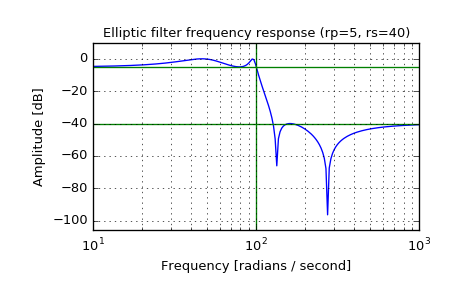# scipy.signal.ellip¶

scipy.signal.ellip(N, rp, rs, Wn, btype='low', analog=False, output='ba')[source]

Elliptic (Cauer) digital and analog filter design.

Design an Nth-order digital or analog elliptic filter and return the filter coefficients.

Parameters: N : int The order of the filter. rp : float The maximum ripple allowed below unity gain in the passband. Specified in decibels, as a positive number. rs : float The minimum attenuation required in the stop band. Specified in decibels, as a positive number. Wn : array_like A scalar or length-2 sequence giving the critical frequencies. For elliptic filters, this is the point in the transition band at which the gain first drops below -rp. For digital filters, Wn is normalized from 0 to 1, where 1 is the Nyquist frequency, pi radians/sample. (Wn is thus in half-cycles / sample.) For analog filters, Wn is an angular frequency (e.g. rad/s). btype : {‘lowpass’, ‘highpass’, ‘bandpass’, ‘bandstop’}, optional The type of filter. Default is ‘lowpass’. analog : bool, optional When True, return an analog filter, otherwise a digital filter is returned. output : {‘ba’, ‘zpk’, ‘sos’}, optional Type of output: numerator/denominator (‘ba’), pole-zero (‘zpk’), or second-order sections (‘sos’). Default is ‘ba’. b, a : ndarray, ndarray Numerator (b) and denominator (a) polynomials of the IIR filter. Only returned if output='ba'. z, p, k : ndarray, ndarray, float Zeros, poles, and system gain of the IIR filter transfer function. Only returned if output='zpk'. sos : ndarray Second-order sections representation of the IIR filter. Only returned if output=='sos'.

Notes

Also known as Cauer or Zolotarev filters, the elliptical filter maximizes the rate of transition between the frequency response’s passband and stopband, at the expense of ripple in both, and increased ringing in the step response.

As rp approaches 0, the elliptical filter becomes a Chebyshev type II filter (cheby2). As rs approaches 0, it becomes a Chebyshev type I filter (cheby1). As both approach 0, it becomes a Butterworth filter (butter).

The equiripple passband has N maxima or minima (for example, a 5th-order filter has 3 maxima and 2 minima). Consequently, the DC gain is unity for odd-order filters, or -rp dB for even-order filters.

The 'sos' output parameter was added in 0.16.0.

Examples

Plot the filter’s frequency response, showing the critical points:

>>> from scipy import signal
>>> import matplotlib.pyplot as plt

>>> b, a = signal.ellip(4, 5, 40, 100, 'low', analog=True)
>>> w, h = signal.freqs(b, a)
>>> plt.semilogx(w, 20 * np.log10(abs(h)))
>>> plt.title('Elliptic filter frequency response (rp=5, rs=40)')
>>> plt.xlabel('Frequency [radians / second]')
>>> plt.ylabel('Amplitude [dB]')
>>> plt.margins(0, 0.1)
>>> plt.grid(which='both', axis='both')
>>> plt.axvline(100, color='green') # cutoff frequency
>>> plt.axhline(-40, color='green') # rs
>>> plt.axhline(-5, color='green') # rp
>>> plt.show()#### Previous topic

scipy.signal.cheb2ord

#### Next topic

scipy.signal.ellipord